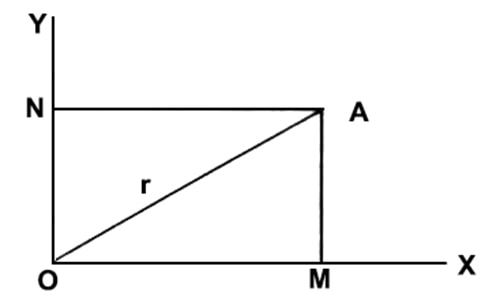# Two Dimensional Reference Frame

Two dimensional reference frame:

Suppose a particle is on a plane and let the particle be in motion. So, its position will be different at different times. In order to designate its position two mutually perpendicular straight lines are needed. OX and OY in the figure are two such straight lines. These two lines have met at O. So, O is the reference point or origin. Here OX is called X-axis and OY is called Y-axis. A frame has been formed with this origin and the two axes. It is called two dimensional reference frame [Figure].Let the particle be at position A at a particular time. From point “A” a normal AM is drawn on OX and another normal AN is drawn on OY. So, OM = AN = x; AM = ON = y. Here the position of A is designated by two co-ordinates x and y. In other words, A is a point whose co-ordinate is x and y. Hence, different particles of a body have two co-ordinates; the body is called two dimensional body.

Let join, OA. If OA = r, there will be many points C, D, E, F etc. on the plane whose distance, from the point O, will be r.

Examples: A moving football in a field is in motion in two dimensional space. Thin paper, thin metal sheet etc. are two dimensional objects.